Select Page

# Vector Algebra MCQ CBSE Maths 12 Science Answers in Hindi

Vector Algebra MCQ CBSE Maths 12 Science Answers in Hindi to enable students to get Answers in a narrative video format for the specific question.

Expert Teacher provides Vector Algebra MCQ CBSE Maths 12 Science Answers through Video Answers in Hindi language. This video solution will be useful for students to understand how to write an answer in exam in order to score more marks. This teacher uses a narrative style for a question from Vector Algebra not only to explain the proper method of answering question, but deriving right answer too.

Please find the question below and view the Answer in a narrative video format.

Question:

## Similar Questions from CBSE, 12th Science, Maths, Vector Algebra

Question 1 :  Find the sum of the vectors :(View Answer Video)

Question 2 : Find a vector in the direction ofthat has magnitude 7 units.   (View Answer Video)

Question 3 :  Write a vector in the direction of the vectorthat has magnitude 9 units.   (View Answer Video)

Question 4 :  Find the sum of the vectorsand(View Answer Video)

Question 5 : Find a vector in the direction of vectorwhich has magnitude 21 units.  (View Answer Video)

### Inverse Trigonometric Functions

Question 1 :is equal to :

### Continuity and Differentiability

Question 1 : If x and y are connected parametrically by the equation, without eliminating the parameter, find. (View Answer Video)

Question 2 : Differentiate the function w.r.t.x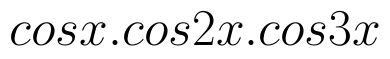. (View Answer Video)

Question 3 : Differentiate the functionwith respect to x. (View Answer Video)

Question 4 : If x and y are connected parametrically by the equation, without eliminating the parameter, find. (View Answer Video)

Question 5 : Findfor the function. (View Answer Video)

### Probability

Question 1 : If E and F are two events such that,, Find P(not E and not F). (View Answer Video)

Question 2 : If P(A)=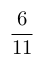, P(B)=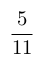and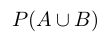=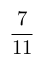, find. (View Answer Video)

Question 3 : Compute P(A/B) if P(B) =0.5 and P(A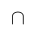B)=0.32. (View Answer Video)

Question 4 : If P(A)=0.8, P(B)=0.5 and=0.4, find. (View Answer Video)